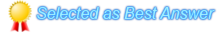You must Sign In to post a response.
• # How to Calculate Decimal values of All Rows From a Table in C#

hi Developers ,

i want to know How to Calculate Decimal values of All Rows From a Table in C# .
already i have done following query

SqlConnection con = Connection.DBConn();
DataTable dt = new DataTable();
SqlCommand cmd = new SqlCommand("[select * from Sales where Totalsales='"Ses_Sales""']", con);
SqlDataAdapter da = new SqlDataAdapter(cmd);
da.Fill(dt);
string sum_QuantityDespatched = dt.Compute("Sum(quantitydespatched)", "").ToString();

above query is working fine. But the problem is it is only working with Integer values.
if the values of table have 3 rows
Value = 1=10
Value = 2=20.
Value = 3=30
i got the my expected output is like = 60:

but if i give the decimal values like this
Value = 1=10.50
Value = 2=20.70
Value = 30=30.00
My expected output is 61.20 . but it throws error.
i want to calculate all rows of a table with Decimal Values . How can i done this task .
anyone know how to done this task please suggest me

Thanking You
Paul.S
•Hi,

Why are you struggling with c# code, simply do it in sql server side that give exact info without any issue's.

For ex if you want to calculate the total sum of the records then use Cummulative sum concept in sql server.

Ex:
`select id, value, sum(value) OVER( order by Id) as cumulativeSum from yourtable`

the above query will give output as like below
`id value cumulativeSum1 10.5 10.52 12.3 22.83 8.5 31.3`

You can simply use the field cumulative-sum when you want to show the running total value.
Hope this helps you....

--------------------------------------------------------------------------------
Give respect to your work, Instead of trying to impress your boss.

N@veen
Blog : http://naveens-dotnet.blogspot.in/

•Hi,

Make sure you don't have null values inside 'quantitydespatched' column.
Also try this:
`Object sum_QuantityDespatched = dt.Compute("Sum(quantitydespatched)", "") ;Console.Write(Convert.ToDecimal(sum_QuantityDespatched));`

• You can try this code snippet in your resource to Calculate Decimal values of All Rows From a Table in C#
` SqlCommand cmd = new SqlCommand("[select Sum(isnull(quantitydespatched,'0')) from Sales where Totalsales='"Ses_Sales""']", con);`

Reference Resources :
http://stackoverflow.com/questions/21619975/how-to-set-decimal-value-in-a-dataset-in-c

http://www.c-sharpcorner.com/forums/how-to-calculate-decimal-values-of-all-rows-from-a-table-in

http://stackoverflow.com/questions/13952812/datarow-is-zeroising-the-decimal-part-of-a-decimal-when-updating-datatable

•You can try the below by changing the string to decimal and remove the .Tostring() at end

decimal sum_QuantityDespatched = Convert.ToDecimal(dt.Compute("Sum(quantitydespatched)", String.Empty));

• i got exact output friends .

howecer thanks a lot for all your valuable reply dear friends and brothers

by

Paul.S

• Sign In to post your comments